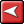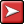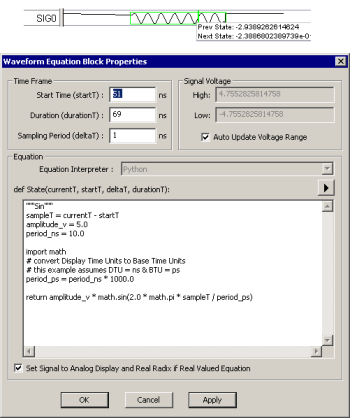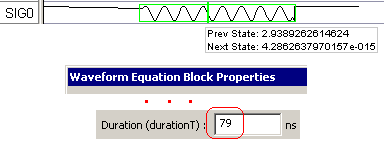## (TD) 4.3 Writing Python Waveform Equation Blocks## (TD) 4.3 Writing Python Waveform Equation BlocksIf you use the pre-defined waveform equation blocks, you will edit the code to control the different parameters like period or amplitude. You can also choose to write your own equations.

Edit the default Sine Wave:

 •Double click on the equation block to open the Waveform Block Properties dialog. If the Edit Bus State dialog opens instead, then press the Edit Block button to get to the Waveform Block Properties dialog.•The State equation is called to calculate the value for each point on the waveform.•The points are at (startT + n * deltaT). It stops when durationT is reached. •Changing the Start Time is the equivalent of dragging and dropping the leading edge of the block's selection box. •Making the Sampling Period smaller will create more points in the block (e.g. durationT/deltaT points will be created by the block).•Make the sine wave cover a longer period of time by adding 10ns to the Duration and pressing the Apply button.Investigate the Code:

 •The first line is a comment string that defines the name of the function. It does not have to be included. """Sin"""
 •Here sampleT is defined as a relative time from the start of the block so that the waveform shape is independent of the start time of the block. sampleT = currentT - startT
 • These lines will normally be edited to control the period and amplitude of the signal. •Change the period to 5.0 and press the Apply button to see the period change. amplitude_v = 5.0 period_ns = 10.0•This imports the standard Python math library so you do not have to define what basic math functions like sin do. import math
 •You must keep track of the time units that you are working on. # convert Display Time Units to Base Time Units # this example assumes DTU = ns & BTU = ps period_ps = period_ns * 1000.0

return amplitude_v * math.sin(2.0 * math.pi * sampleT / period_ps)

 •In the last line, a real value is returned for the current sample point. The math.sin( ) function is the sine function in the Python math library.